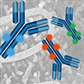# Product Results## Primary Antibody Conjugates

Choose from a wide range of directly conjugated primary antibodies, validated and optimized for your specific application. Not seeing the one you want? Let CST conjugate it for you.

# Product Name Application Reactivity
H, M, R, Hm, Mk, Dm, Sc
H, M, R, Hm, Mk, Mi, Dm, Z, B, Dg, Pg, Sc
H, M, R, Mk, Sc
H, M, R, Mk, Sc
H, M, R, Mk, Sc
H, M, R, Mk, Dm, Sc
H, M, R, Mk, Dm, Sc
H, M, R, Mk, Dm, Sc
H, M, R, Mk, Mi, Pg, Sc
H, M, R, Mk, Dm, Pg, Sc
H, M, R, Mk, Dm, Pg, Sc
H, M, R, Mk, Dm, Pg, Sc
H, M, R, Mk, Sc
H, M, R, Mk, Dm, Pg, Sc
H, M, R, Mk, Dm, Pg, Sc
H, M, R, Mk, Dm, Pg, Sc
H, M, R, Mk, Sc
H, M, R, Mk, Mi, Sc
H, M, R, Mk, Mi, Sc
H, M, R, Mk, Mi, Sc
H, M, R, Mk, Mi, Sc
H, M, R, Mk, Mi, Sc
H, M, R, Mk, Mi, Sc
H, M, R, Mk, Mi, Sc
H, M, R, Mk, Mi, Sc
H, M, R, Mk, Mi, Sc
H, M, R, Mk, Mi, Sc
H, M, R, Hm, Sc
H, M, R, Hm, Sc